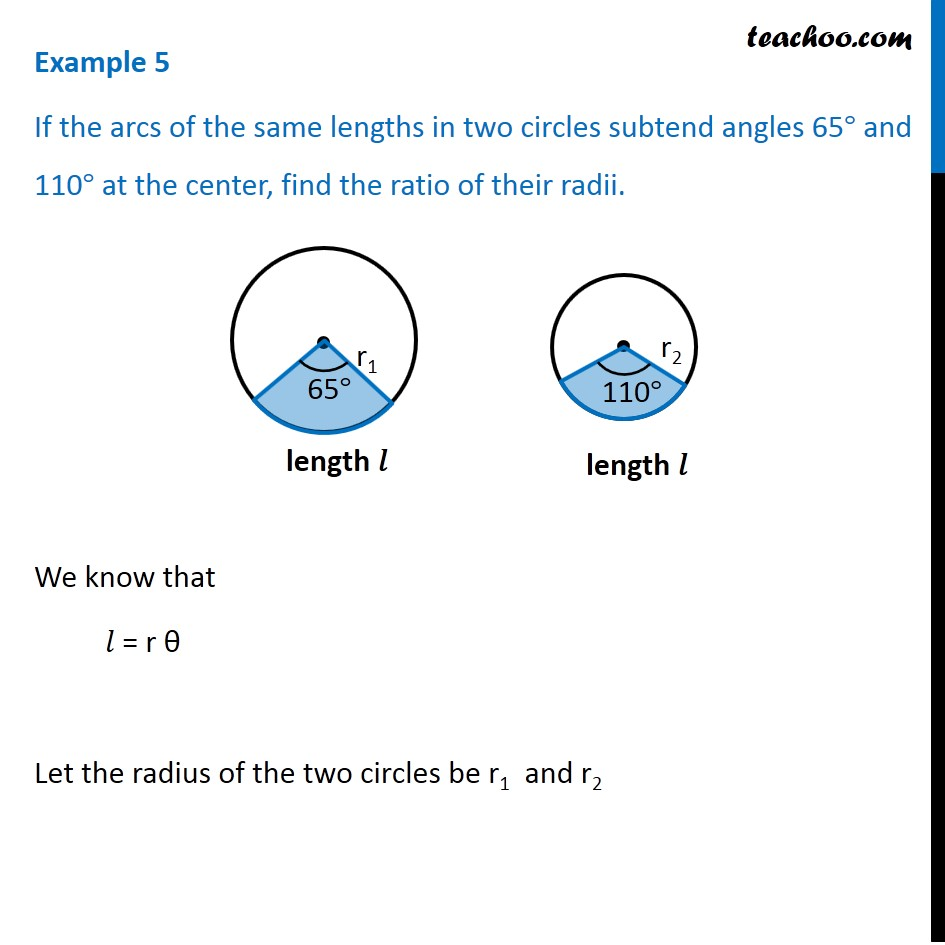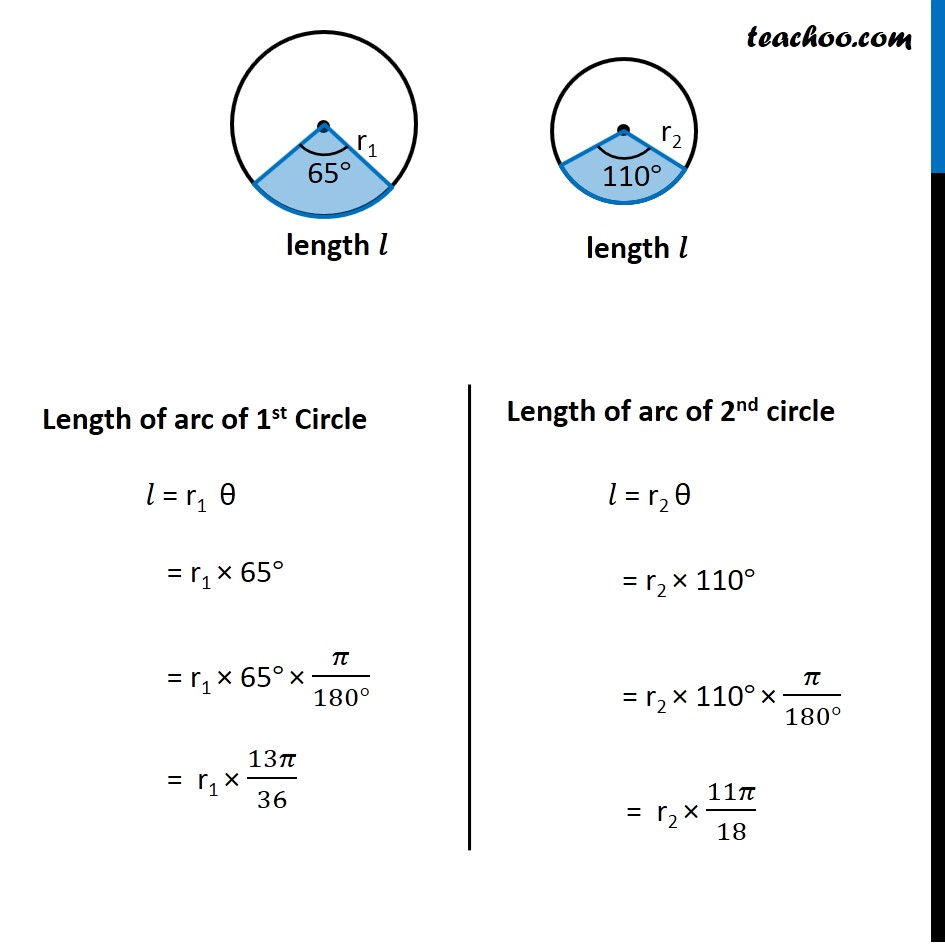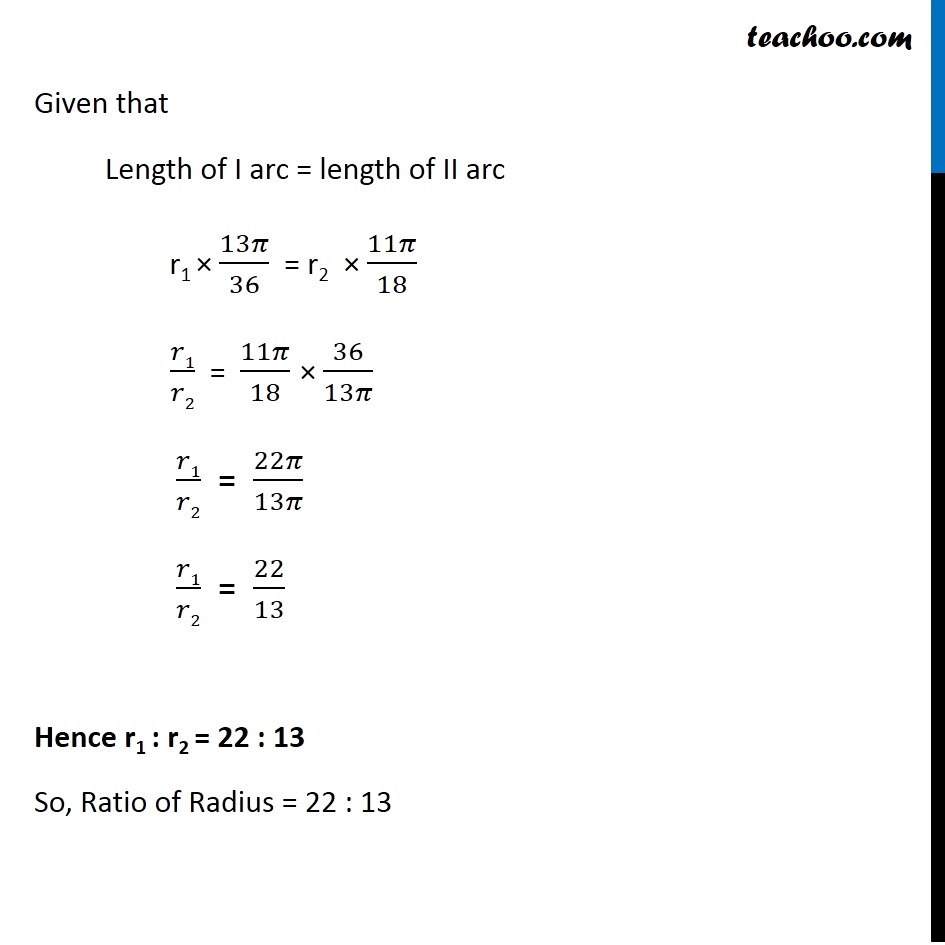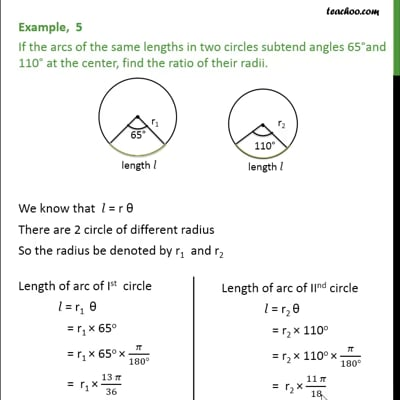Examples

Chapter 3 Class 11 Trigonometric Functions
Serial order wiseThis video is only available for Teachoo black users

Solve all your doubts with Teachoo Black (new monthly pack available now!)

### Transcript

Example 5 If the arcs of the same lengths in two circles subtend angles 65° and 110° at the center, find the ratio of their radii. We know that 𝑙 = r θ Let the radius of the two circles be r1 and r2 Length of arc of 1st Circle 𝑙 = r1 θ = r1 × 65° = r1 × 65° × 𝜋/(180°) = r1 × 13𝜋/36 Length of arc of 2nd circle 𝑙 = r2 θ = r2 × 110° = r2 × 110° × 𝜋/(180°) = r2 × 11𝜋/18 Given that Length of I arc = length of II arc r1 × 13𝜋/36 = r2 × 11𝜋/18 𝑟1/𝑟2 = 11𝜋/18 × 36/13𝜋 𝑟1/𝑟2 = 22𝜋/13𝜋 𝑟1/𝑟2 = 22/13 Hence r1 : r2 = 22 : 13 So, Ratio of Radius = 22 : 13